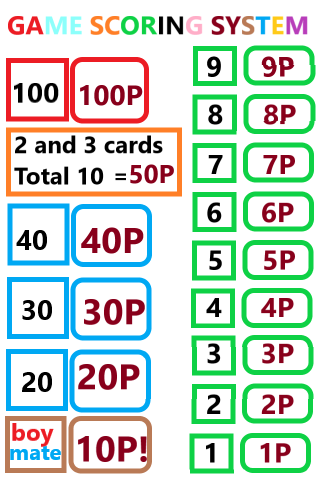###### Ranking and Scoring in Boymate10

In Boymate10 there are special combinations of cards' value and scoring. The smallest of the cards is 1 and the largest is 100 in the scoreboard. In the game consisting of 54 cards, there are geometric cards with 13 scores on four colors. Unlike these, there are 2 more cards named boymate10, which gave the game that completed 50 points, while the other card with 10 points alone was 10 points.

One of the basic rules that are effective in calculating the points of the game is the rule of collecting the points of the same kind of cards. Here, while boymate10 completes the small card to 50 points, if the color of the other card is the same, the total score is calculated by adding 50.

For example, if the score value of the two cards in blue is 1 and 8, the boymate10 card is also discarded.

The card with a value of 1 combines with the boymate10 and becomes 50 points, additionally added at 8 points, and a total of 50 + 8 hand points becomes 58 points.

If the color of the cards is different, there will be 50p in total if there is boymate10. This rule takes place when you do not have a 100 point card. If the color of the other card is different when there is 100 points, the boymate10 will be 10 points and the total will be 110 points, while the color of the card will be 100 + 50 = 150 points.

For example :

When 100 yellow cards and 1 blue card and boymate10 = 110 points;

100 yellow cards and 1 yellow card and boymate10 = 150 points.

There is no need to memorize them one by one. It is enough to try to make a strategy using the same breeds. And it is necessary to know that boymate10 has two point values.

Attempting to find bonuses that give the game the skeleton task of the game requires establishing strategy and thinking in the flow of the game. When the total point value of the 2 or 3 cards selected is 10, they form a whole and the total point value becomes 50 points. If the total value of the two cards is 10 and the same color in the other card, 50 points are added with the score value of the other card.

For example: When 3 Blue and 7 Blue and 9 Blue are thrown, 3 and 7 = 50 points and 9 points total 50 + 9 = 59 points are obtained.

While trying to get high points and leaving high card point values ​​to the future hands, it is tried to predict the future card and get high points from the opponent. In each hand consisting of 9 hands, each hand wins 50 points, except for the card points.

The last hand is a surprise, and the hand score is 150 points.

At the end of the game, additional points are calculated beside the points in the leaderboard.

• ### Don't stop, download now! Discover boymate10 with its new design.